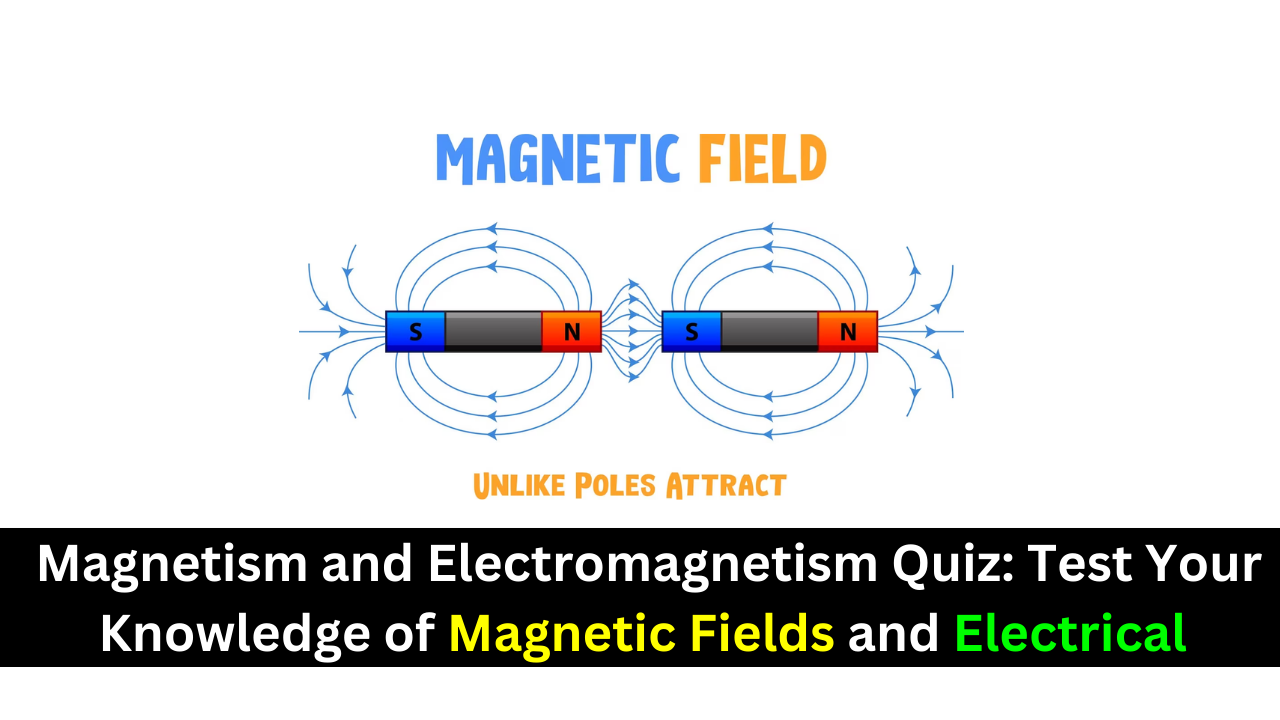Thursday, October 26

## Magnetism and Electromagnetism Quiz of Magnetic Fields and Electrical Principles### When the south poles of two bar magnets are brought close together, there will be

(a) a force of attraction
(b) a force of repulsion
(c) an upward force
(d) no force
Answer: (b) a force of repulsion

### A magnetic field is made up of

(a) positive and negative charges
(b) magnetic domains
(c) flux lines
(d) magnetic poles

### The direction of a magnetic field is from

(a) north pole to south pole
(b) south pole to north pole
(c) inside to outside the magnet
(d) front to back
Answer: (a) north pole to south pole

### Reluctance in a magnetic circuit is analogous to

(a) voltage in an electric circuit
(b) current in an electric circuit
(c) power in an electric circuit
(d) resistance in an electric circuit
Answer: (d) resistance in an electric circuit

(a) tesla
(b) weber
(c) gauss
(d) ampere-turn

### The unit of magnetomotive force is the

(a) tesla
(b) weber
(c) ampere-turn
(d) electron-volt

### A unit of magnetic flux density is the

(a) tesla
(b) weber
(c) ampere-turn
(d) ampere-turns/meter

### The electromagnetic activation of a movable shaft is the basis for

(a) relays
(b) circuit breakers
(c) magnetic switches
(d) solenoids

### When there is current through a wire placed in a magnetic field,

(a) the wire will overheat
(b) the wire will become magnetized
(c) a force is exerted on the wire
(d) the magnetic field will be canceled
Answer: (c) a force is exerted on the wire

### A coil of wire is placed in a changing magnetic field. If the number of turns in the coil is increased, the voltage

nduced across the coil will
(a) remain unchanged
(b) decrease
(c) increase
(d) be excessive

### If a conductor is moved back and forth at a constant rate in a constant magnetic field, the voltage induced in the conductor will

(a) remain constant
(b) reverse polarity
(c) be reduced
(d) be increased

### In the crankshaft position sensor in Figure 34, the induced voltage across the coils is caused by

(a) current in the coil
(b) rotation of the disk
(c) a tab passing through the magnetic field
(d) acceleration of the disk’s rotational speed
Answer: (b) rotation of the disk

### The purpose of the commutator in a generator or motor is to

(a) change the direction of the current to the rotor as it spins
(b) change the direction of the current to the stator windings
(c) support the motor or generator shaft
(d) provide the magnetic field for the motor or generator
Answer: (a) change the direction of the current to the rotor as it spins

### In a motor, back emf serves to

(a) increase the power from the motor
(b) decrease the flux
(c) increase the current in the field windings
(d) decrease the current in the armature
Answer: (d) decrease the current in the armature

### The torque of a motor is proportional to the

(a) amount of flux
(b) armature current
(c) both of the above
(d) none of the above
Answer: (c) both of the above

### The difference between alternating current (ac) and direct current (dc) is

(a) ac changes value and dc does not
(b) ac changes direction and dc does not
(c) both (a) and (b)
(d) neither (a) nor (b)
Answer: (b) ac changes direction and dc does not

### During each cycle, a sine wave reaches a peak value

(a) one time
(b) two times
(c) four times
(d) a number of times depending on the frequency

(a) 20 kHz
(b) 15,000 Hz
(c) 10,000 Hz
(d) 1.25 MHz

### A sine wave with a period of 2 ms is changing at a faster rate than a sine wave with a period of

(a) 1 ms
(b) 0.0025 s
(c) 1.5 ms
(d) (Not provided in the options)

(a) 6 cycles
(b) 10 cycles
(c) 1/16 cycle
(d) 600 cycles

### If the peak value of a sine wave is 10 V, the peak-to-peak value is

(a) 20 V
(b) 5 V
(c) 100 V
(d) none of these

(a) 14.14 V
(b) 6.37 V
(c) 7.07 V
(d) 0.707 V

(a) 0 V
(b) 6.37 V
(c) 7.07 V
(d) 5 V

(a) 0 V
(b) 6.37 V
(c) 12.74 V
(d) 14.14 V

### If the rms current through a resistor is 5 mA, the rms voltage drop across the resistor is

(a) 70.7 V
(b) 7.07 V
(c) 5 V
(d) 50 V

An advantage of a three-phase induction motor is that it

(a) maintains constant speed for any load
(b) does not require starting windings
(c) has a wound rotor
(d) all of the above
Answer: (d) all of the above

### The difference in the synchronous speed of the stator field and the rotor speed of a motor is called

(a) differential speed
(c) lag
(d) slip

### A 10 kHz pulse waveform consists of pulses that are wide. Its duty cycle is

(a) 100%
(b) 10%
(c) 1%
(d) not determinable

### The duty cycle of a square wave

(a) varies with the frequency
(b) varies with the pulse width
(c) both (a) and (b)
(d) is 50%
Answer: (c) both (a) and (b)

Related Posts

Share.
error: Content is protected !!